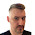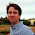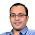Dec 30, 2012

Coin Puzzle: Predict the Other's Coin

Source: Puzzle collection by Raphael ReischukProblem:
Assume the following 3-player game consisting of several rounds. Players A and B build a team, they have one fair coin each, and may initially talk to each other. Before starting the first round, however, no more communication between them is allowed until the end of the game. (Imagine they are separated in different places without any communication infrastructure.)

A round of the game consists of the following steps:
(1) the team gives one dollar to player C.
(2) Both A and B toss their coins independently.
(3) Both A and B try to predict the other's coin by telling the guess to C. (No communication: A does not know the outcome of B's coin toss, and vice versa, nor the guess).
(4) If C verifies that both A and B guess the other's coin correctly, then C has to give 3 dollars back to the team.

Should C play this game?

Previously Asked Coin Puzzles:
Coin Balancing
Coins Puzzle
Five Thieves and Bounty

Update:
Discussion on Hacker News: http://news.ycombinator.com/item?id=4985537

Update: (29 Jan 2013)
Correct solution by: Takaki, Andre, Felix, JDGM in comments!
Correct strategy (but not so correct calculation) by Joshua, Shubham Mittal in comments!
If you are just looking for the solution, Perfect solution by Andre. Thanks

1.No. A and B can win with probability 1/2 by saying heads if their own coin is heads and tails if their coin is tails. Then both will guess correctly if the outcome is HH or TT.

2.No,
A and B guess the same as their own coin toss.
A B GuessA GuessB Win
H H H H Yes
H T H T No
T H T H No
T T T T Yes
Expected Value for C = (2*1 - 2*3)/4 = -\$1 per round

3.Why not? He has a 50/50 chance of profiting 2 dollars and only stands to lose 1 dollar if the team gueses wrong.

4.C should play, it's a fun game. Also the expected value of their winnings is 25c, but C should be happy with the risk that they might lose. If the game can be played repeatedly C can expect to make a profit in the long run.

Let A be the event that A guesses correctly; B that B guesses correctly. P(A) = P(B) = 1/2

Events A and B are independent (no communication) so
P(A and B) = P(A) x P(B) = 1/4
P(neither A nor B) = 1 - P(A and B) = 3/4

Expected value of C's winnings:
P(A and B) x (\$1 - \$3) + P(neither A nor B) x \$1
= 1/4 x -\$2 + 3/4 x \$1
= 25c

5.Its 50-50 ... for loss-gain odds

6.in point 1, did you mean each team give C a dollar? mean total is 2 dollars?

7.in point 4, did u mean C has to give back 3 dollars to each team (A & B). so total is \$6?

8.yes, even in the event that C is an honest player. A has 1/2 chances of being correct and B has 1/2 chances of being correct. this is a total of 1/4 chances of both being correct. Each round C gains 1 dollar minus 3 dollars multiplied by the chance of A and B being correct (1/4). so C leaves each round with 1 - (3/4) = 1/4. on average C should gain 25 cents each round (1/4 of a dollar). i've been out of school for a while am i missing something obvious?

9.Consider the following strategy. Both A and B always guess that the result of the coin toss of the other player is the same as theirs (so e.g. if A gets heads, they guess that B also got heads). With two independent coin flips there is 0.5 probability that the two coins are either both heads or both tails. In that case A and B would both guess correctly, so therefore they have a 0.5 chance of winning and 0.5 probability of losing.

So with probability 0.5 player C will earn 1 dollar and with probability 0.5 player C will lose 2 dollars (1 gained initially -3 given away in the end). Then the expected value of the game for C will be
E = 0.5*1 + 0.5*(-2) = 0.5 - 1 = -0.5

Therefore if A and B are smart players (and decide on a good strategy like always guessing the same or always guessing the opposite of what they get) then C should NOT play this game.

(Note that if A and B would guess randomly each time, then the probability that they would get each others coin toss results correct would be 0.5 * 0.5 = 0.25 and the expected value for C would be
E = 0.25*(-2) + 0.75*1 = -0.5 + 0.75 = 0.25,
so then C SHOULD play the game)

10.Probability for C to win is 3/4.

Hence the expectation for C is 0.

So he should not play

11.How does the game end? Is it A and B who choose went to stop playing?

12.C should not play this game.
A and B can win with probability one half, simply by guessing that the other has the same toss (e.g., if A obtains tail, he guesses that B has tail as well, and vice-versa). Since both tosses are equal with probability one half, the expecte gain of C is
1 - 3 * 1/2 = -1/2
which is negative, so he should not play.

13.C should play,

as making a correct predict for a team is 1/2, it makes that C will loose(if both predict right)with probability with 1/4.

so out of 4 matches C looses 1 and wins 3.
for wining 3 he get Rs3
and for loosing 1 he gets Rs 1 but then give back Rs3, so total loos of Rs2 in this match.
Finally after 4 matches C have Rs3-Rs2=Rs1.

1.You din't take in account the fact that "A & B may initially talk to each other".
This will allow them to make some strategy to guess correct answer with higher probability.
e.g.
Their strategy could be "They guess the same as their own coin toss."
A (Guess by A) B (Guess by B) Win
H H H H Yes
H H T T No
T T H H No
T T T T Yes
So now this strategy increases their winning probability to 0.5
so expected gain of C per round = 1*0.5-3*0.5 = -\$1

14.Nice one.

If A and B agree always to say what they see on their own coin:

Tails-Tails: C loses \$3.
Tails-Heads: C wins \$1.
Heads-Tails: C wins \$1.

These outcomes are equally likely so C's expected return per round is negative \$0.5. Ouch! Don't play!

Note: If A and B agree to say the opposite of what they see on their own coin then it works the same.

15.Typo: "C loses \$3" should be "C loses \$2" in both places I wrote it. This is because C takes \$1 from the players at the start.

I did realise this when calculating my expectation of negative \$0.5, just typed it wrong in the table.

16.Great. We all agree that C should not play.

Some of you are missing the point that "A and B can initially talk to each other"
Some of you are calculating the payoff wrong. If C wins, it has 1 dollar. If C loses, it has to give 1-3=-2 dollars.

The strategy that A and B follow:
A and B will just say the coin that they have. Hence, on HH and TT - they win. Otherwise, they lose. So, half probability C would win and half that C would lose. Since, C loses more when he loses but gains less when he wins, C should not play.

Correct solution by: Takaki, Andre, Felix, JDGM
Correct strategy (but not so correct calculation) by Joshua, Shubham Mittal

If you are just looking for the solution, Perfect solution by Andre. Thanks

17.Expected pay off for C is 1/2 -1/2*2 which is negative. So C should never play

Fraction Brainteaser

Source: Sent to me by Gaurav Sinha Problem: Siddhant writes a Maths test and correctly answers 5 out of 6 Arithmetic questions and 20...